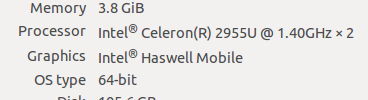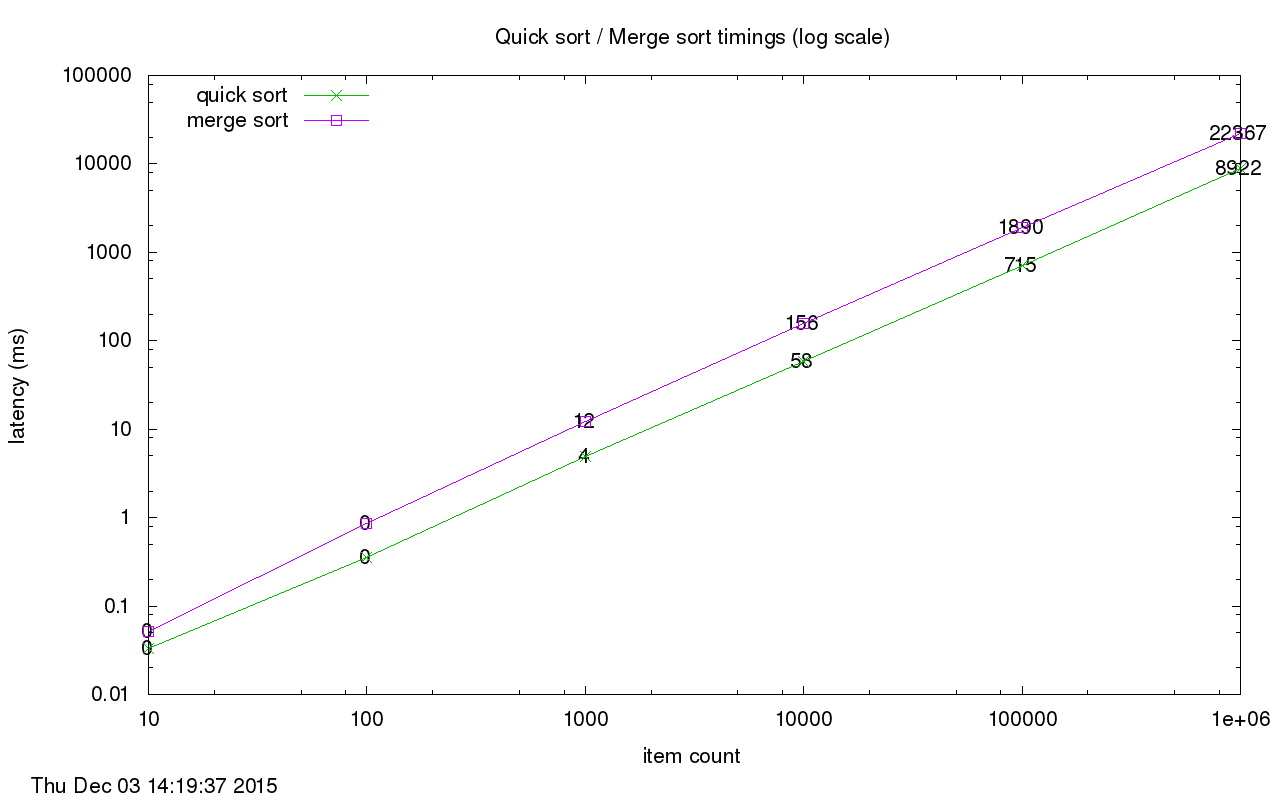# Two Sorts With Rust

Here are some initial thoughts on Rust in the almost two years since I last looked at it along with some implementations of merge and quick sort. (These are just my opinions so please don’t panic !)
1. Cargo is awesome for managing package dependencies and building projects.
2. Rust is a very nice systems programming language that supports a functional style of programming. Much easier to work with than C/C++ with very near the same performance in many cases.
3. Strong static inferred type system !
4. The authors have not neglected the rich and varied history of programming language theory while trying to be very pragmatic about the design and usefulness of the language.
Let’s look at implementing some popular sorting algorithms. First … quick sort.
I won’t be pedantic here by explaining the quick sort algorithm but an elegant and performant implementation is ~20 LOC. Not bad for a systems level programming language.

```pub fn quicksort_rec(nums: Vec) -> Vec {
return match nums.len() {
cnt if cnt <= 1 => nums,
cnt => {
let mut left = Vec::new();
let mut right = Vec::new();
let pivot = nums;
for i in 1..cnt {
match nums[i] {
num if num < pivot => left.push(num),
num => right.push(num),
}
}
let mut left_sorted = quicksort_rec(left);
let mut right_sorted = quicksort_rec(right);
left_sorted.push(pivot);
left_sorted.append(&mut right_sorted);
return left_sorted;
},
};
}
```

An implementation of merge sort is a bit longer at ~35 LOC.

```fn merge(mut left: Vec, mut right: Vec) -> Vec {
let mut merged = Vec::new();
while !left.is_empty() && !right.is_empty() {
if left.last() >= right.last() {
merged.push(left.pop().unwrap());
} else {
merged.push(right.pop().unwrap());
}
}
while !left.is_empty() {
merged.push(left.pop().unwrap());
}
while !right.is_empty() {
merged.push(right.pop().unwrap());
}
merged.reverse();
return merged;
}
pub fn mergesort_rec(nums: Vec) -> Vec {
return match nums.len() {
cnt if cnt <= 1 => nums,
cnt => {
let mut left = Vec::new();
let mut right = Vec::new();
let middle = cnt / 2;
for i in (0..middle).rev() { left.push(nums[i]); }
for i in (middle..cnt).rev() { right.push(nums[i]); }
left = mergesort_rec(left);
right = mergesort_rec(right);
return merge(left, right);
},
};
}
```

Lastly, here are the timings for my very CPU under-powered laptop …The code for the project can be found here.